# Bayesian nonparametrics in NIMBLE: Density estimation

NIMBLE provides functionality for fitting models involving Dirichlet process priors using either the Chinese Restaurant Process (CRP) or a truncated stick-breaking (SB) representation of the Dirichlet process prior.

Here we illustrate NIMBLE’s BNP capabilities by showing how to use nonparametric mixture models with different kernels for density estimation. In a later post, we will take a parametric generalized linear mixed model and show how to switch it to a nonparametric representation of the random effects that avoids the assumption of normally-distributed random effects.

## Basic density estimation using Dirichlet Process Mixture models

NIMBLE provides the machinery for nonparametric density estimation by means of Dirichlet process mixture (DPM) models (Ferguson, 1974; Lo, 1984; Escobar, 1994; Escobar and West, 1995). For an independent and identically distributed sample $$y_1, \ldots, y_n$$, the model takes the form

$y_i \mid \theta_i \sim p(y_i \mid \theta_i), \quad\quad \theta_i \mid G \sim G, \quad\quad G \mid \alpha, H \sim \mbox{DP}(\alpha, H), \quad\quad i=1,\ldots, n .$

The NIMBLE implementation of this model is flexible and allows for mixtures of arbitrary kernels, $$p(y_i \mid \theta)$$, which can be either conjugate or non-conjugate to the (also arbitrary) base measure $$H$$. In the case of conjugate kernel / base measure pairs, NIMBLE is able to detect the presence of the conjugacy and use it to improve the performance of the sampler.

To illustrate these capabilities, we consider the estimation of the probability density function of the waiting time between eruptions of the Old Faithful volcano data set available in R.

data(faithful)

The observations $$y_1, \ldots, y_n$$ correspond to the second column of the dataframe, and $$n = 272$$.

## Fitting a location-scale mixture of Gaussian distributions using the CRP representation

### Model specification

We first consider a location-scale Dirichlet process mixture of normal distributionss fitted to the transformed data $$y_i^{*} = \log (y_i)$$:

$y^{*}_i \mid \mu_i, \sigma^2_i \sim \mbox{N}(\mu_i, \sigma^2_i), \quad (\mu_i, \sigma^2_i) \mid G \sim G, \quad G \mid \alpha, H \sim \mbox{DP}(\alpha, H), \quad i=1,\ldots, n,$

where $$H$$ corresponds to a normal-inverse-gamma distribution. This model can be interpreted as providing a Bayesian version of kernel density estimation for $$y^{*}_i$$ using Gaussian kernels and adaptive bandwidths. On the original scale of the data, this translates into an adaptive log-Gaussian kernel density estimate.

Introducing auxiliary variables $$\xi_1, \ldots, \xi_n$$ that indicate which component of the mixture generates each observation, and integrating over the random measure $$G$$, we obtain the CRP representation of the model (Blackwell and MacQueen, 1973):

$y_i^{*} \mid \{ \tilde{\mu}_k \}, \{ \tilde{\sigma}_k^{2} \} \sim \mbox{N}\left( \tilde{\mu}_{\xi_i}, \tilde{\sigma}^2_{\xi_i} \right), \quad\quad \xi \mid \alpha \sim \mbox{CRP}(\alpha), \quad\quad (\tilde{\mu}_k, \tilde{\sigma}_k^2) \mid H \sim H, \quad\quad i=1,\ldots, n ,$

where

$p(\xi \mid \alpha) = \frac{\Gamma(\alpha)}{\Gamma(\alpha + n)} \alpha^{K(\xi)} \prod_k \Gamma\left(m_k(\xi)\right),$

$$$K(\xi) \le n$$ is the number of unique values in the vector $$\xi$$, and $$m_k(\xi)$$ is the number of times the $$k$$-th unique value appears in $$\xi$$. This specification makes it clear that each observation belongs to any of at most $$n$$ normally distributed clusters, and that the CRP distribution corresponds to the prior distribution on the partition structure. NIMBLE’s specification of this model is given by library(nimble, warn.conflicts = FALSE) ## nimble version 1.0.1 is loaded. ## For more information on NIMBLE and a User Manual, ## please visit https://R-nimble.org. ## ## Note for advanced users who have written their own MCMC samplers: ## As of version 0.13.0, NIMBLE's protocol for handling posterior ## predictive nodes has changed in a way that could affect user-defined ## samplers in some situations. Please see Section 15.5.1 of the User Manual. code <- nimbleCode({ for(i in 1:n) { y[i] ~ dnorm(mu[i], var = s2[i]) mu[i] <- muTilde[xi[i]] s2[i] <- s2Tilde[xi[i]] } xi[1:n] ~ dCRP(alpha, size = n) for(i in 1:n) { muTilde[i] ~ dnorm(0, var = s2Tilde[i]) s2Tilde[i] ~ dinvgamma(2, 1) } alpha ~ dgamma(1, 1) }) Note that in the model code the length of the parameter vectors muTilde and s2Tilde has been set to $$n$$. We do this because the current implementation of NIMBLE requires that the length of vector of parameters be set in advance and does not allow for their number to change between iterations. Hence, if we are to ensure that the algorithm always performs as intended we need to work with the worst case scenario, i.e., the case where there are as many components as observations. While this ensures that the algorithm always works as intended, it is also somewhat inefficient, both in terms of memory requirements (when $$n$$ is large a large number of unoccupied components need to be maintained) and in terms of computational burden (a large number of parameters that are not required for posterior inference need to be updated at every iteration). When we use a mixture of gamma distributions below, we will show a computational shortcut that improves the efficiency. Note also that the value of $$\alpha$$ controls the number of components we expect a priori, with larger values of $$\alpha$$ corresponding to a larger number of components occupied by the data. Hence, by assigning a prior to $$\alpha$$ we add flexibility to the model specification. The particular choice of a Gamma prior allows NIMBLE to use a data-augmentation scheme to efficiently sample from the corresponding full conditional distribution. Alternative prior specifications for $$\alpha$$ are possible, in which case the default sampler for this parameter is an adaptive random-walk Metropolis-Hastings algorithm. ### Running the MCMC algorithm The following code sets up the data and constants, initializes the parameters, defines the model object, and builds and runs the MCMC algorithm. Because the specification is in terms of a Chinese restaurant process, the default sampler selected by NIMBLE is a collapsed Gibbs sampler (Neal, 2000). set.seed(1) # Model Data lFaithful <- log(faithful$waiting)
standlFaithful <- (lFaithful - mean(lFaithful)) / sd(lFaithful)
data <- list(y = standlFaithful)
# Model Constants
consts <- list(n = length(standlFaithful))
# Parameter initialization
inits <- list(xi = sample(1:10, size=consts$n, replace=TRUE), muTilde = rnorm(consts$n, 0, sd = sqrt(10)),
s2Tilde = rinvgamma(consts$n, 2, 1), alpha = 1) # Model creation and compilation rModel <- nimbleModel(code, data = data, inits = inits, constants = consts)  ## Defining model ## Building model ## Setting data and initial values ## Running calculate on model ## [Note] Any error reports that follow may simply reflect missing values in model variables. ## Checking model sizes and dimensions cModel <- compileNimble(rModel)  ## Compiling ## [Note] This may take a minute. ## [Note] Use 'showCompilerOutput = TRUE' to see C++ compilation details. # MCMC configuration, creation, and compilation conf <- configureMCMC(rModel, monitors = c("xi", "muTilde", "s2Tilde", "alpha"))  ## ===== Monitors ===== ## thin = 1: alpha, muTilde, s2Tilde, xi ## ===== Samplers ===== ## CRP_concentration sampler (1) ## - alpha ## CRP_cluster_wrapper sampler (544) ## - s2Tilde[] (272 elements) ## - muTilde[] (272 elements) ## CRP sampler (1) ## - xi[1:272] mcmc <- buildMCMC(conf) cmcmc <- compileNimble(mcmc, project = rModel)  ## Compiling ## [Note] This may take a minute. ## [Note] Use 'showCompilerOutput = TRUE' to see C++ compilation details. samples <- runMCMC(cmcmc, niter = 7000, nburnin = 2000, setSeed = TRUE)  ## running chain 1... ## |-------------|-------------|-------------|-------------| ## |-------------------------------------------------------| We can extract the samples from the posterior distributions of the parameters and create trace plots, histograms, and any other summary of interest. For example, for the concentration parameter $$\alpha$$ we have: # Trace plot for the concentration parameter ts.plot(samples[ , "alpha"], xlab = "iteration", ylab = expression(alpha))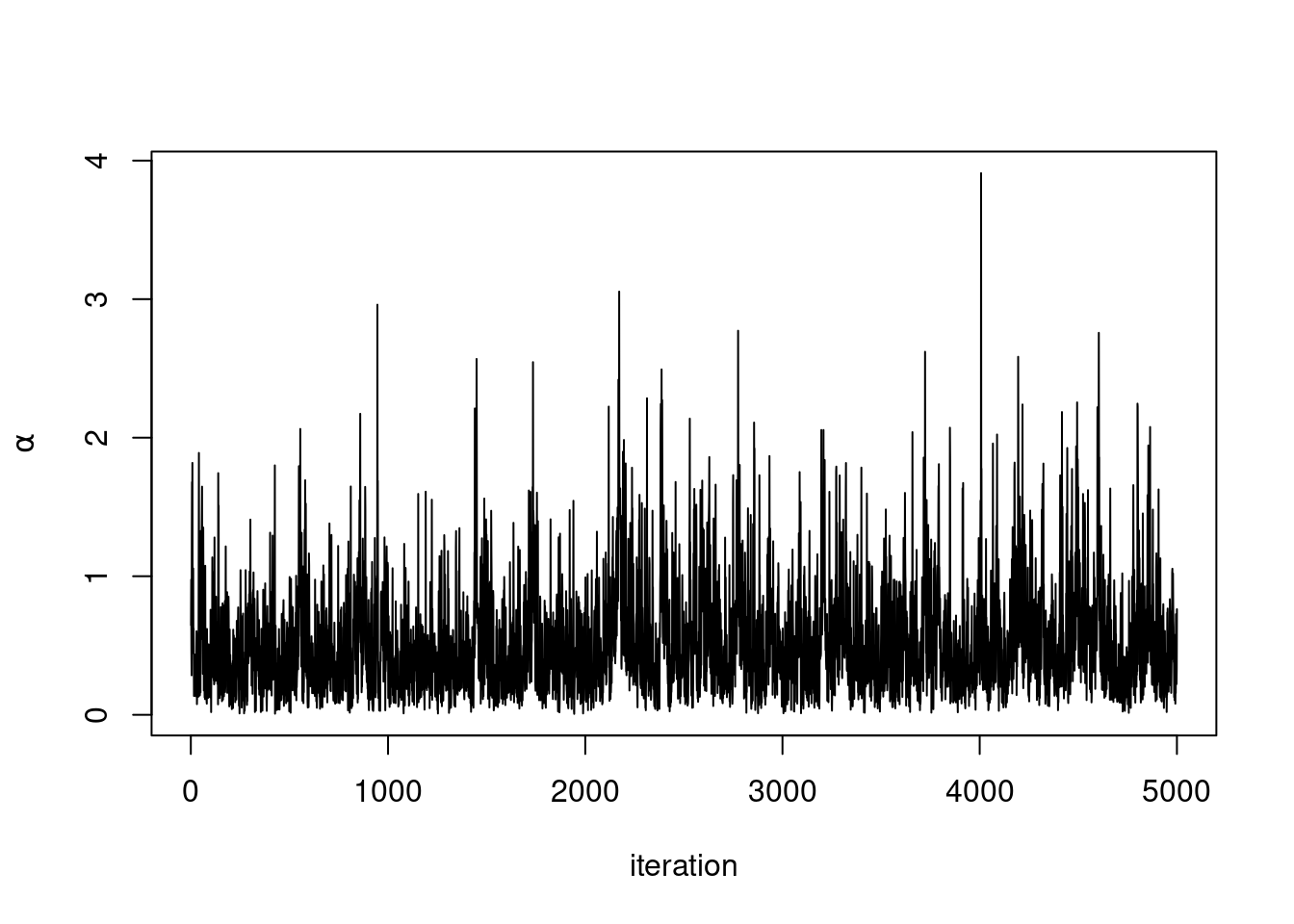# Posterior histogram hist(samples[ , "alpha"], xlab = expression(alpha), main = "", ylab = "Frequency")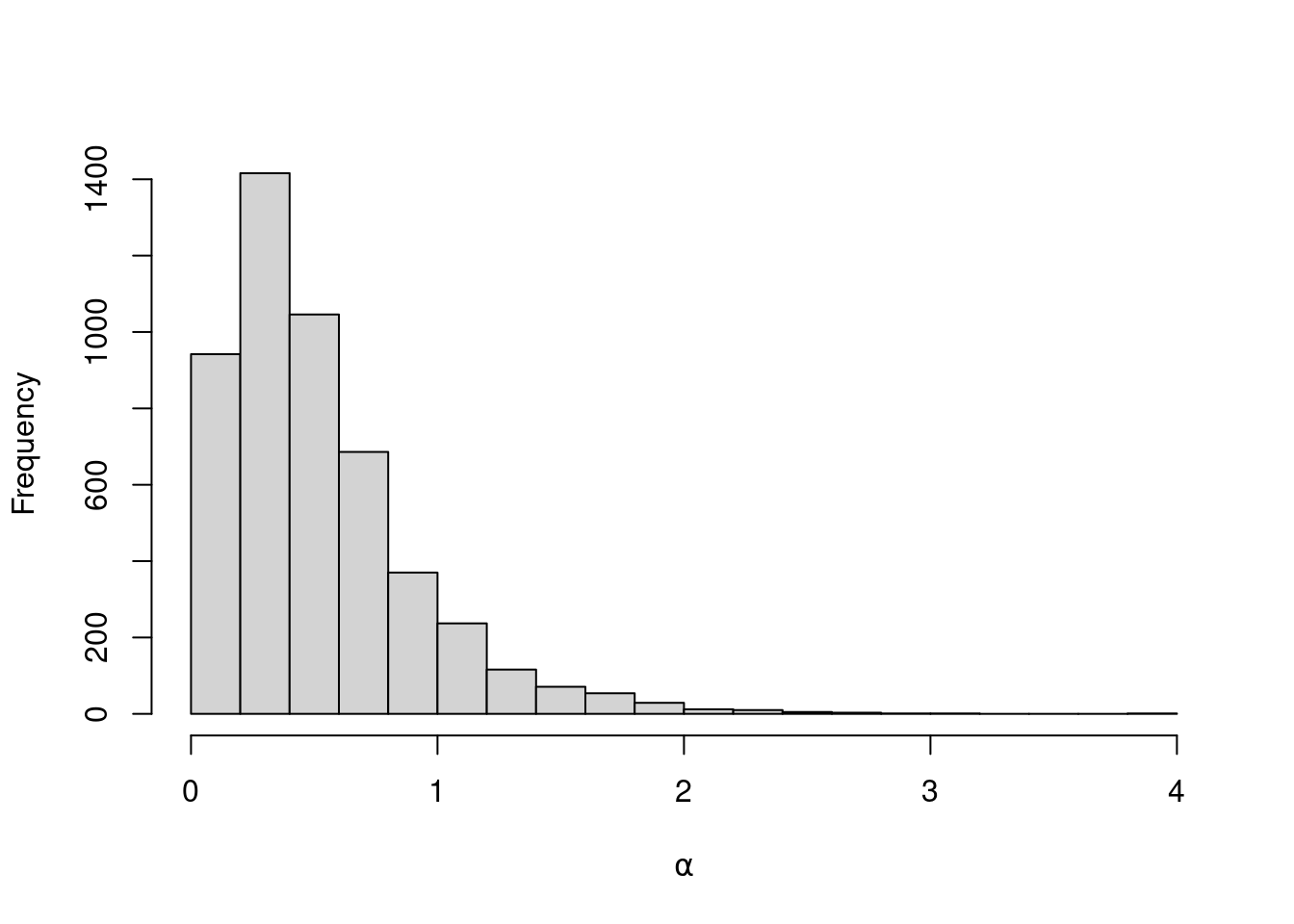quantile(samples[ , "alpha"], c(0.5, 0.025, 0.975)) ## 50% 2.5% 97.5% ## 0.4230550 0.0580579 1.5608958 Under this model, the posterior predictive distribution for a new observation $$\tilde{y}$$, $$p(\tilde{y} \mid y_1, \ldots, y_n)$$, is the optimal density estimator (under squared error loss). Samples for this estimator can be easily computed from the samples generated by our MCMC: # posterior samples of the concentration parameter alphaSamples <- samples[ , "alpha"] # posterior samples of the cluster means muTildeSamples <- samples[ , grep('muTilde', colnames(samples))] # posterior samples of the cluster variances s2TildeSamples <- samples[ , grep('s2Tilde', colnames(samples))] # posterior samples of the cluster memberships xiSamples <- samples [ , grep('xi', colnames(samples))] standlGrid <- seq(-2.5, 2.5, len = 200) # standardized grid on log scale densitySamplesStandl <- matrix(0, ncol = length(standlGrid), nrow = nrow(samples)) for(i in 1:nrow(samples)){ k <- unique(xiSamples[i, ]) kNew <- max(k) + 1 mk <- c() li <- 1 for(l in 1:length(k)) { mk[li] <- sum(xiSamples[i, ] == k[li]) li <- li + 1 } alpha <- alphaSamples[i] muK <- muTildeSamples[i, k] s2K <- s2TildeSamples[i, k] muKnew <- muTildeSamples[i, kNew] s2Knew <- s2TildeSamples[i, kNew] densitySamplesStandl[i, ] <- sapply(standlGrid, function(x)(sum(mk * dnorm(x, muK, sqrt(s2K))) + alpha * dnorm(x, muKnew, sqrt(s2Knew)) )/(alpha+consts$n))
}

hist(data$y, freq = FALSE, xlim = c(-2.5, 2.5), ylim = c(0,0.75), main = "", xlab = "Waiting times on standardized log scale") ## pointwise estimate of the density for standardized log grid lines(standlGrid, apply(densitySamplesStandl, 2, mean), lwd = 2, col = 'black') lines(standlGrid, apply(densitySamplesStandl, 2, quantile, 0.025), lty = 2, col = 'black') lines(standlGrid, apply(densitySamplesStandl, 2, quantile, 0.975), lty = 2, col = 'black')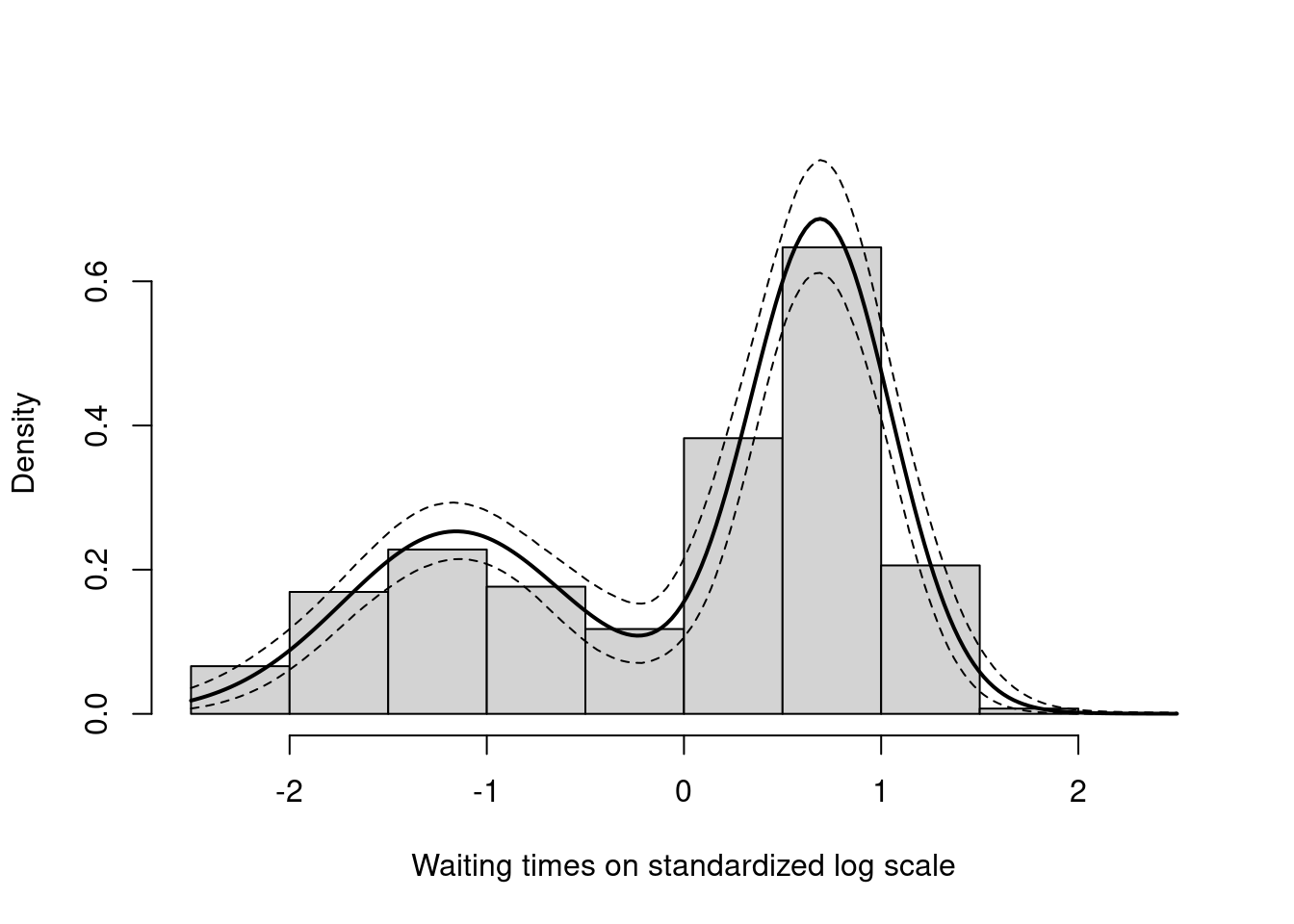Recall, however, that this is the density estimate for the logarithm of the waiting time. To obtain the density on the original scale we need to apply the appropriate transformation to the kernel. lgrid <- standlGrid*sd(lFaithful) + mean(lFaithful) # grid on log scale densitySamplesl <- densitySamplesStandl / sd(lFaithful) # density samples for grid on log scale hist(faithful$waiting, freq = FALSE, xlim = c(40, 100), ylim=c(0, 0.05),
main = "", xlab = "Waiting times")
lines(exp(lgrid), apply(densitySamplesl, 2, mean)/exp(lgrid), lwd = 2, col = 'black')
lines(exp(lgrid), apply(densitySamplesl, 2, quantile, 0.025)/exp(lgrid), lty = 2,
col = 'black')
lines(exp(lgrid), apply(densitySamplesl, 2, quantile, 0.975)/exp(lgrid), lty = 2,
col = 'black')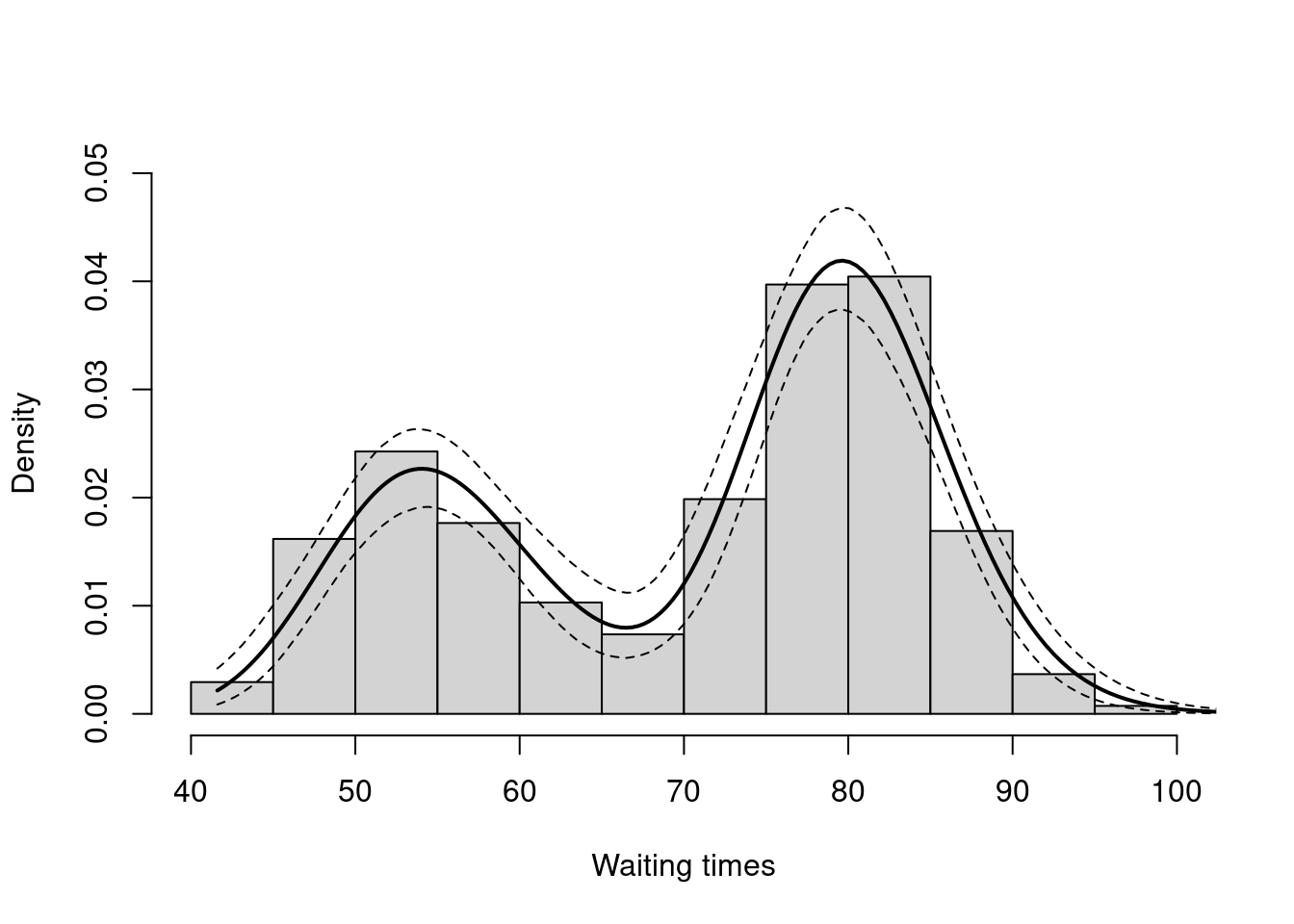In either case, there is clear evidence that the data has two components for the waiting times.

### Generating samples from the mixing distribution

While samples from the posterior distribution of linear functionals of the mixing distribution $$G$$ (such as the predictive distribution above) can be computed directly from the realizations of the collapsed sampler, inference for non-linear functionals of $$G$$ requires that we first generate samples from the mixing distribution. In NIMBLE we can get posterior samples from the random measure $$G$$, using the getSamplesDPmeasure function. Note that, in order to get posterior samples from $$G$$, we need to monitor all the random variables involved in its computations, i.e., the membership variable, xi, the cluster parameters, muTilde and s2Tilde, and the concentration parameter, alpha.

The following code generates posterior samples from the random measure $$G$$. The cMCMC object includes the model and posterior samples from the parameters. The getSamplesDPmeasure function estimates a truncation level of $$G$$, namely truncG. The posterior samples are in a matrix with $$(\mbox{truncG} \cdot (p+1))$$ columns, where $$p$$ is the dimension of the vector of parameters with distribution $$G$$ (in this example $$p=2$$).

samplesG <- getSamplesDPmeasure(cmcmc) 
## Compiling
##   [Note] This may take a minute.
##   [Note] Use 'showCompilerOutput = TRUE' to see C++ compilation details.
## |-------------|-------------|-------------|-------------|
## |-------------------------------------------------------|

The following code computes posterior samples of $$P(\tilde{y} > 70)$$ using the posterior samples from the random measure $$G$$. Note that these samples are computed based on the transformed model and a value larger than 70 corresponds to a value larger than 0.03557236 on the above defined grid.

weightIndex <- grep('weight', colnames(samplesG[]))
muTildeIndex <- grep('muTilde', colnames(samplesG[]))
s2TildeIndex <- grep('s2Tilde', colnames(samplesG[]))

probY70 <- rep(0, nrow(samples))  # posterior samples of P(y.tilde > 70)
for(i in seq_len(nrow(samples))) {
probY70[i] <- sum(samplesG[[i]][, weightIndex] *
pnorm(0.03557236, mean = samplesG[[i]][, muTildeIndex],
sd = sqrt(samplesG[[i]][, s2TildeIndex]), lower.tail = FALSE))
}

hist(probY70,  xlab = "Probability", ylab = "P(yTilde > 70 | data)" , main = "" )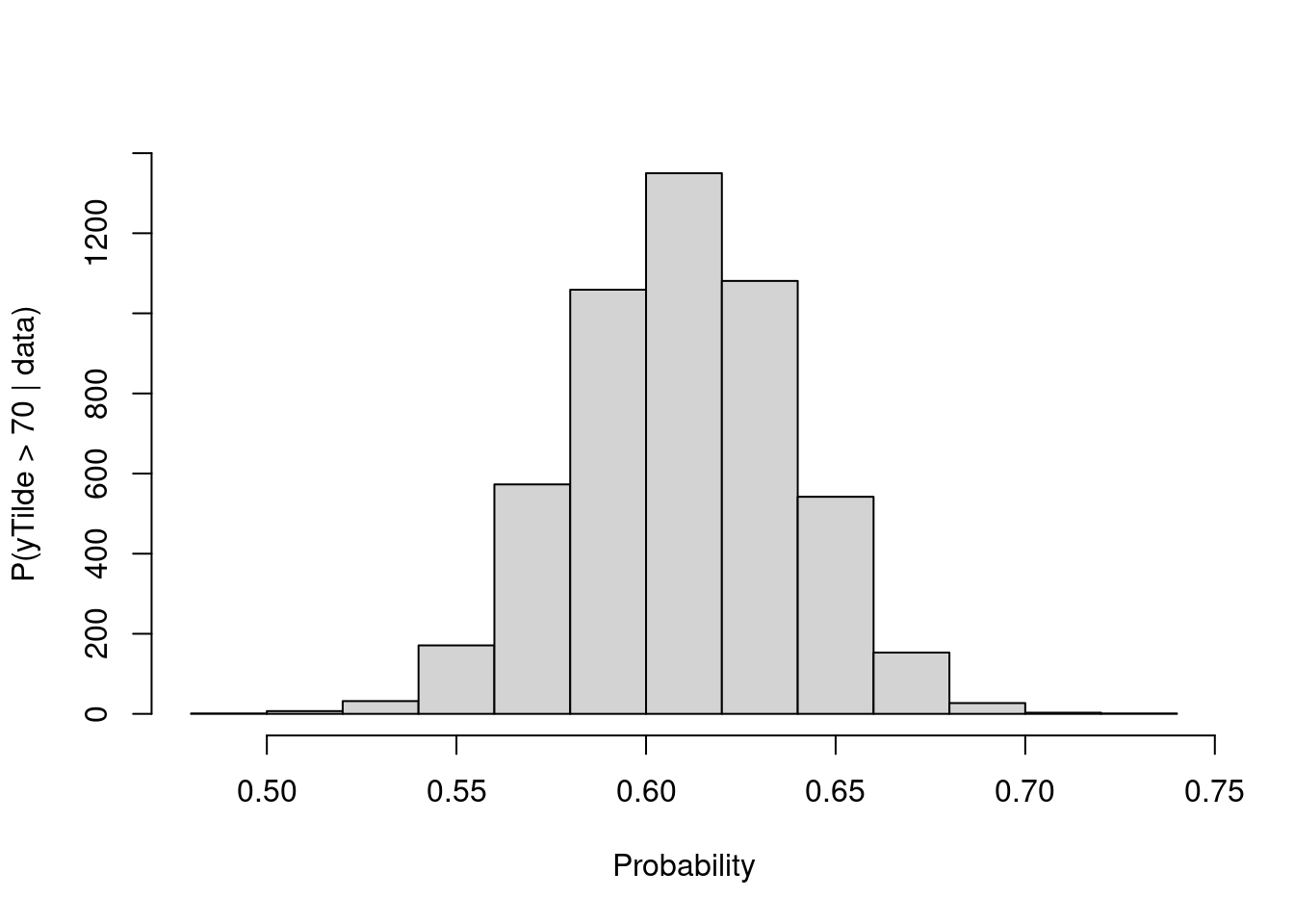## Fitting a mixture of gamma distributions using the CRP representation

NIMBLE is not restricted to using Gaussian kernels in DPM models. In the case of the Old Faithful data, an alternative to the mixture of Gaussian kernels on the logarithmic scale that we presented in the previous section is a (scale-and-shape) mixture of Gamma distributions on the original scale of the data.

### Model specification

In this case, the model takes the form

$y_i \mid \{ \tilde{\beta}_k \}, \{ \tilde{\lambda}_k \} \sim \mbox{Gamma}\left( \tilde{\beta}_{\xi_i}, \tilde{\lambda}_{\xi_i} \right), \quad\quad \xi \mid \alpha \sim \mbox{CRP}(\alpha), \quad\quad (\tilde{\beta}_k, \tilde{\lambda}_k) \mid H \sim H ,$

where $$H$$ corresponds to the product of two independent Gamma distributions. The following code provides the NIMBLE specification for the model:

code <- nimbleCode({
for(i in 1:n) {
y[i] ~ dgamma(shape = beta[i], scale = lambda[i])
beta[i] <- betaTilde[xi[i]]
lambda[i] <- lambdaTilde[xi[i]]
}
xi[1:n] ~ dCRP(alpha, size = n)
for(i in 1:50) { # only 50 cluster parameters
betaTilde[i] ~ dgamma(shape = 71, scale = 2)
lambdaTilde[i] ~ dgamma(shape = 2, scale = 2)
}
alpha ~ dgamma(1, 1)
})

Note that in this case the vectors betaTilde and lambdaTilde have length $$50 \ll n = 272$$. This is done to reduce the computational and storage burdens associated with the sampling algorithm. You could think about this approach as truncating the process, except that it can be thought of as an exact truncation. Indeed, under the CRP representation, using parameter vector(s) with a length that is shorter than the number of observations in the sample will lead to a proper algorithm as long as the number of components instatiated by the sampler is strictly lower than the length of the parameter vector(s) for every iteration of the sampler.

### Running the MCMC algorithm

The following code sets up the model data and constants, initializes the parameters, defines the model object, and builds and runs the MCMC algorithm for the mixture of Gamma distributions. Note that, when building the MCMC, a warning message about the number of cluster parameters is generated. This is because the lengths of betaTilde and lambdaTilde are smaller than $$n$$. Also, note that no error message is generated during execution, which indicates that the number of clusters required never exceeded the maximum of 50.

data <- list(y = faithful$waiting) set.seed(1) inits <- list(xi = sample(1:10, size=consts$n, replace=TRUE),
betaTilde = rgamma(50, shape = 71, scale = 2),
lambdaTilde = rgamma(50, shape = 2, scale = 2),
alpha = 1)
rModel <- nimbleModel(code, data = data, inits = inits, constants = consts)
## Defining model
## Building model
## Setting data and initial values
## Running calculate on model
##   [Note] Any error reports that follow may simply reflect missing values in model variables.
## Checking model sizes and dimensions
cModel <- compileNimble(rModel)
## Compiling
##   [Note] This may take a minute.
##   [Note] Use 'showCompilerOutput = TRUE' to see C++ compilation details.
conf <- configureMCMC(rModel, monitors = c("xi", "betaTilde", "lambdaTilde", "alpha"))
## ===== Monitors =====
## thin = 1: alpha, betaTilde, lambdaTilde, xi
## ===== Samplers =====
## CRP_concentration sampler (1)
##   - alpha
## CRP_cluster_wrapper sampler (100)
##   - betaTilde[]  (50 elements)
##   - lambdaTilde[]  (50 elements)
## CRP sampler (1)
##   - xi[1:272]
mcmc <- buildMCMC(conf)
##   [Warning] sampler_CRP: The number of clusters based on the cluster parameters is less than the number of potential clusters. The MCMC is not strictly valid if it ever proposes more components than cluster parameters exist; NIMBLE will warn you if this occurs.
cmcmc <- compileNimble(mcmc, project = rModel)
## Compiling
##   [Note] This may take a minute.
##   [Note] Use 'showCompilerOutput = TRUE' to see C++ compilation details.
samples <- runMCMC(cmcmc, niter = 7000, nburnin = 2000, setSeed = TRUE)
## running chain 1...
## |-------------|-------------|-------------|-------------|
## |-------------------------------------------------------|

In this case we use the posterior samples of the parameters to construct a trace plot and estimate the posterior distribution of $$\alpha$$:

# Trace plot of the posterior samples of the concentration parameter
ts.plot(samples[ , 'alpha'], xlab = "iteration", ylab = expression(alpha))